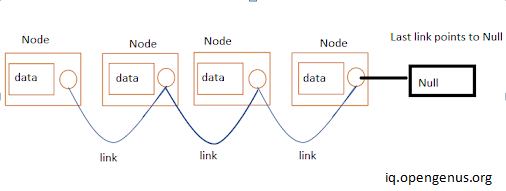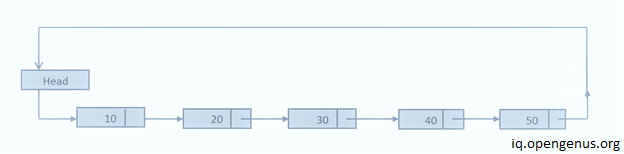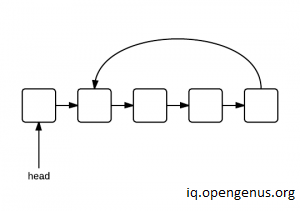×

Search anything:

# To check if the linked list is a circular linked list (2 methods)

#### Algorithms linked list Data StructuresIndian Technical Authorship Contest starts on 1st July 2023. Stay tuned.

We will be seeing two efficient methods to determine whether the given linked list is a circular linked list or not. Before Coding , Let's review basic terminologies inorder to get familiar with the problem .

Linked list is a linear data structure where each element called node, is a separate object.Unlike Arrays,the elements are not necessarily stored next to each other but store each other's memory addresses through pointers.Linked list's each node is divided into two parts :

1. Contains data or information of element.
2. Also called linkfield or nextpointer field which contains nodes of the next node of the list.What is a circular linked list?Circular linked list is a variation of linked list where the last node is pointing to the first node's information part.Therefore the last node does not point to null.

Circular list vs list with loop• Circular linked list is entirely circular and the last node points to the first or the head node.
• A loop exists in a LinkedList when no NULL is reached as we traverse throughout the LinkedList.

How might we identify whether the given linked list is circular?

When we traverse entire linked list:

• If any node seems to be pointing towards the head or starting node then the linked list is circular.
• If no node is pointing to null.

Then it means that the linked list is circular in nature.

###### Algorithm to find whether the given linked list is circular

Method-1
A very simple way to determine whether the linked list is circular or not

2. Check if the node is pointing to the head.
3. If yes then it is circular.
Let's look at the snippet where we code this algorithm.

#### Pseudocode

``````* Create a structure for linked list
Declare:
-Variable to store data of node.
-Pointer variable of struct type to store address of next node.

* function of datatype bool isCircular(firstNode){

-Store the value of first node in temp variable and make it traverse all nodes.
-temp=firstNode
-temp=next node pointed by temp(temp->next)
-run until temp is at null or firstNode
if (temp at null)
not circular and returns false.
if (temp points first node)
return true as its circular.
}

* function of datatype node newNode(data){

-To insert new nodes and link each one of them to the previous node
by storing the address of the new node to the previous one.
-Then make them point to NULL.
}

* In int main function

-First insert nodes for circular linked list and check its nature by calling isCircular function.
-Since it is true through if statement it prints "yes".
-Second insert a normal linked list and check its nature by calling isCircular function.
As its not circular it prints "no".
``````
``````#include <iostream>
using namespace std;
//node structure
struct node{
int data;
struct node* next;
};
//function to find the circular linked list.
while(temp!=NULL)
{ //if temp points to head then it has completed a circle,thus a circular linked list.
return true;
temp=temp->next;
}

return false;

}
//function for inserting new nodes.
node *newNode(int data){
struct node *temp=new node;
temp->data=data;
temp->next=NULL;
}
int main() {
//first case
cout<<"yes"<<endl;
else
cout<<"no"<<endl;
//second case

cout<<"yes";
else
cout<<"no";
return 0;
}

``````
``````//output
Yes
No
``````

### Output Explanation:

#### First Example

Two elements were inserted and then the second one was pointed to the first one.

``````struct node* head=newNode(1);//How list is now: 1->NULL
\________/
``````

They have been inserted using the newNode function.

``````node *newNode(int data){
struct node *temp=new node;//Node created
temp->data=data;//Data inserted e.g: 1
temp->next=NULL;//1->NULL
}
``````

Now if statement is evaluated.The list looks like:

``````// List is 1->2->3->4
//         \_______/
``````

When if statement is evaluated , it processes isCircular function and the list undergoes following steps:

``````(in isCircular function)
while(temp!=NULL) // never reach null as list is circlular.
{
return true;//so returns true
temp=temp->next;//from 1 it moves to 2 then to 3 and so on.
}
``````

Based on true or false returned it prints yes or no respectively.

``````
cout<<"yes"<<endl;// if statement is true so prints "yes"
else
cout<<"no"<<endl;
``````

Time Complexity:O(n)

Method-2 (Floyd's Algorithm)
Also called Tortoise and hare algorithm : Suppose you have a tortoise and a hare running in a circular path.Hare being the faster one is going to meet tortoise at some point of the time.Similarly , if a linked list is circular and two pointers(slow and fast) are traversing the linked list ,then the faster one will meet the slower one at some point.Using this logic we will determine whether the linked list is circular or not.
It is an efficient algorithm with time complexity of O(n) as the worst case is when the faster pointer has traversed the list twice whereas the slower one has hardly completed the traversal once.
And the space complexity too remains constant with O(1).

### Pseudocode:

``````In function of booltype isCircular(firstnode){
1. Declare  two pointers pointing to the first node.
2. Make faster pointer traverse faster.
3. Since the faster pointer is faster than the slow pointer, if it reaches null or points to null ,
then immediately it is known that the linked list is not circular.
4. If the fast pointer meets slow pointer or points to it ,
then it is known that the linked list is circular.}
``````

Now we will code as per the algorithm in C++ :

``````#include <iostream>
using namespace std;

struct node{
int data;
struct node* next;
};
{ //fast ptr is ahead of slow pointer
node* slow,*fast;
//if linked list is empty it returns true as its entirely null.
return true;
while(true)
{ //fast ptr traverses quickly so If its not circular then it reaches or points to null.
if(fast==NULL||fast->next==NULL)
{
return false;
}
//when circular fast meets or points to slow pointer while traversing
else if(slow==fast||fast->next==slow)
{
return true;
}
//for moving forward through linked list.
else
{
slow=slow->next;
//fast traverses way ahead so it distinguishes loops out of circular list.
fast=fast->next->next;
}
}
}

node *newNode(int data){
struct node *temp=new node;
temp->data=data;
temp->next=NULL;
}
int main() {
cout<<"yes"<<endl;
else
cout<<"no"<<endl;

cout<<"yes";
else
cout<<"no";
return 0;
}

``````

Output:

``````Yes
No
``````

### First Case when it is circular linked list:

The function is called in which:
slow pointer is made to point to the head and fast is pointing to the next node.

``````//linked list is: 1->2->12->122
//                 \________/

``````

Now , fast is not null nor its pointing to it and not even it is at slow or pointing to slow.
so

``````//let |x|y| be node where x is data and y is address of next node.
1st iteration:

2nd iteration:(neither fast->next==slow nor fast->next==NULL)

3rd iteration:(neither fast->next==slow nor fast->next==NULL)

4th iteration:

if(fast->next==NULL||fast==NULL) from 4th iteration, fast->next=slow.
return true;
Output: yes
``````

How this approach distinguishes between loop and circular list?

What if it was a looped linked list(eg. 1<->2-3-4 loop in btw 1 and 2)?In here as fast pointer is at 2 and slow at 1.Obviously fast will reach null and return false.
In this manner it eliminates the flaw of counting of looped ones as circular linked lists.

In this way we can determine whether the given linked list is circular or not.

#### Rashmitha

Final Year, B.tech (Information Technology ),Indira Gandhi Delhi Technical University For Women